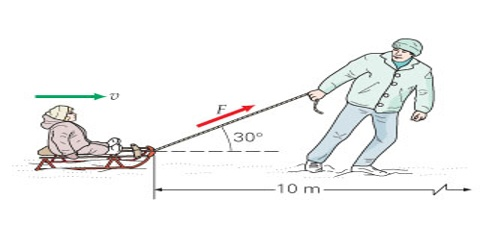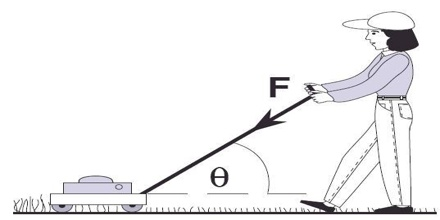Physics

# Work Done by the ForceWork Done by the Force

If a force is applied on a moving football along its direction of motion, then the football moves towards the direction of the force. When a mango falls on the ground from a tree, due to the action of gravitational force it falls directly downward. In both the cases, work is positive or it is understood that work is done by the force. So, it can be said that when the point of action of the applied force moves along the direction of force or if there is a component of the displacement along the direction of the force, then it is understood that work is done by the force. In this case work done by the force is positive work.Work is done whenever a force moves something over a distance. You can calculate the energy transferred, or work done, by multiplying the force by the distance moved in the direction of the force.

Energy transferred = work done = force x distance moved in the direction of the force